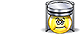Unit Conversion Software Web Widgets Loan Calculator Currency Rates Country Flags Unit Converter
 Faq HelpPressure ConverterContact
 From: To: pascal [Pa]exapascal [EPa]petapascal [PPa]terapascal [TPa]gigapascal [GPa]megapascal [MPa]kilopascal [kPa]hectopascal [hPa]dekapascal [daPa]decipascal [dPa]centipascal [cPa]millipascal [mPa]micropascal [µPa]nanopascal [nPa]picopascal [pPa]femtopascal [fPa]attopascal [aPa]newton/square meter [N/m^2]newton/square centimeter [N/cm^2]newton/square millimeter [N/mm^2]kilonewton/square meter [kN/m^2]barmillibar [mbar]microbar [µbar]dyne/square centimeter [dyn/cm^2]kilogram-force/square meter [kgf/m^2]kilogram-force/square centimeterkilogram-force/square millimetergram-force/square centimeter [gf/cm^2]ton-force (short)/square footton-force (short)/square inchton-force (long)/square footton-force (long)/square inchkip-force/square inch [kipf/in^2]ksi [ksi]pound-force/square foot [lbf/ft^2]pound-force/square inch [lbf/in^2]psi [psi]poundal/square foot [pdl/ft^2]torr [Torr]centimeter mercury (0°C) [cmHg]millimeter mercury (0°C) [mmHg]inch mercury (32°F) [inHg]inch mercury (60°F) [inHg]centimeter water (4°C) [cmAq]millimeter water (4°C) [mmAq]inch water (4°C) [inAq]foot water (4°C) [ftAq]inch water (60°F) [inAq]foot water (60°F) [ftAq]atmosphere technical [at]Standard atmosphere [atm] pascal [Pa]exapascal [EPa]petapascal [PPa]terapascal [TPa]gigapascal [GPa]megapascal [MPa]kilopascal [kPa]hectopascal [hPa]dekapascal [daPa]decipascal [dPa]centipascal [cPa]millipascal [mPa]micropascal [µPa]nanopascal [nPa]picopascal [pPa]femtopascal [fPa]attopascal [aPa]newton/square meter [N/m^2]newton/square centimeter [N/cm^2]newton/square millimeter [N/mm^2]kilonewton/square meter [kN/m^2]barmillibar [mbar]microbar [µbar]dyne/square centimeter [dyn/cm^2]kilogram-force/square meter [kgf/m^2]kilogram-force/square centimeterkilogram-force/square millimetergram-force/square centimeter [gf/cm^2]ton-force (short)/square footton-force (short)/square inchton-force (long)/square footton-force (long)/square inchkip-force/square inch [kipf/in^2]ksi [ksi]pound-force/square foot [lbf/ft^2]pound-force/square inch [lbf/in^2]psi [psi]poundal/square foot [pdl/ft^2]torr [Torr]centimeter mercury (0°C) [cmHg]millimeter mercury (0°C) [mmHg]inch mercury (32°F) [inHg]inch mercury (60°F) [inHg]centimeter water (4°C) [cmAq]millimeter water (4°C) [mmAq]inch water (4°C) [inAq]foot water (4°C) [ftAq]inch water (60°F) [inAq]foot water (60°F) [ftAq]atmosphere technical [at]Standard atmosphere [atm] Result:How to use Pressure Converter
Select the unit to convert from in the input units list. Select the unit to convert to in the output units list. Enter the value to convert from into the input box on the left. The conversion result will immediately appear in the output box.Download Pressure Unit Converter
our powerful software utility that helps you make easy conversion between more than 2,100 various units of measure in more than 70 categories. Discover a universal assistant for all of your unit conversion needs - download the free demo version right away!Copyright © 1998-2009 UnitConversion.org Privacy & Terms | About | Faq | Help | Contact | Link to Us